The NLMIXED Procedure
 Integral Approximations

An important part of the marginal maximum likelihood method described previously is the computation of the integral over the random effects. The default method in PROC NLMIXED for computing this integral is adaptive Gaussian quadrature as described in Pinheiro and Bates (1995). Another approximation method is the first-order method of Beal and Sheiner (1982, 1988). A description of these two methods follows.

A quadrature method approximates a given integral by a weighted sum over predefined abscissas for the random effects. A good approximation can usually be obtained with an adequate number of quadrature points as well as appropriate centering and scaling of the abscissas. Adaptive Gaussian quadrature for the integral over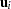centers the integral at the empirical Bayes estimate of, defined as the vector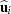that minimizes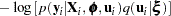with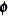and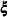set equal to their current estimates. The final Hessian matrix from this optimization can be used to scale the quadrature abscissas.

Suppose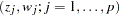denote the standard Gauss-Hermite abscissas and weights (Golub and Welsch 1969, or Table 25.10 of Abramowitz and Stegun 1972). The adaptive Gaussian quadrature integral approximation is as follows: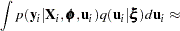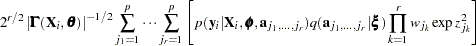whereis the dimension of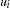,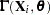is the Hessian matrix from the empirical Bayes minimization,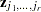is a vector with elements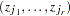, and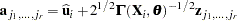PROC NLMIXED selects the number of quadrature points adaptively by evaluating the log-likelihood function at the starting values of the parameters until two successive evaluations have a relative difference less than the value of the QTOL= option. The specific search sequence is described under the QFAC= option. Using the QPOINTS= option, you can adjust the number of quadrature points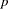to obtain different levels of accuracy. Setting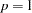results in the Laplacian approximation as described in Beal and Sheiner (1992), Wolfinger (1993), Vonesh (1992, 1996), Vonesh and Chinchilli (1997), and Wolfinger and Lin (1997).

The NOAD option in the PROC NLMIXED statement requests nonadaptive Gaussian quadrature. Here allare set equal to zero, and the Cholesky root of the estimated variance matrix of the random effects is substituted for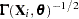in the preceding expression for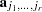. In this case derivatives are computed using the algorithm of Smith (1995). The NOADSCALE option requests the same scaling substitution but with the empirical Bayes.

PROC NLMIXED computes the derivatives of the adaptive Gaussian quadrature approximation when carrying out the default dual quasi-Newton optimization.

### First-Order Method

Another integral approximation available in PROC NLMIXED is the first-order method of Beal and Sheiner (1982, 1988) and Sheiner and Beal (1985). This approximation is used only in the case where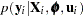is normal—that is,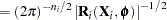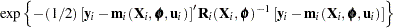where
is the dimension of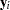,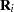is a diagonal variance matrix, and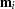is the conditional mean vector of.

The first-order approximation is obtained by expanding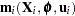with a one-term Taylor series expansion about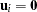, resulting in the approximation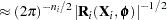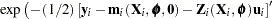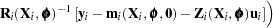where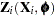is the Jacobian matrix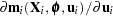evaluated at.

Assuming that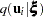is normal with meanand variance matrix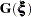, the first-order integral approximation is computable in closed form after completing the square: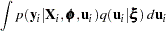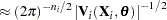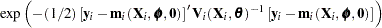where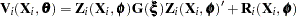. The resulting approximation for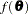is then minimized over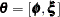to obtain the first-order estimates. PROC NLMIXED uses finite-difference derivatives of the first-order integral approximation when carrying out the default dual quasi-Newton optimization.Previous Page | Next Page | Top of Page